## Cycloconverter:

A variable frequency supply for feeding a three phase motor can be obtained from a cycloconverter which operates on a 50 Hz supply and provides a variable frequency supply at the output (Fig. 3.105). These are single stage frequency conversion devices which make use of both line commutation as well as forced commutation.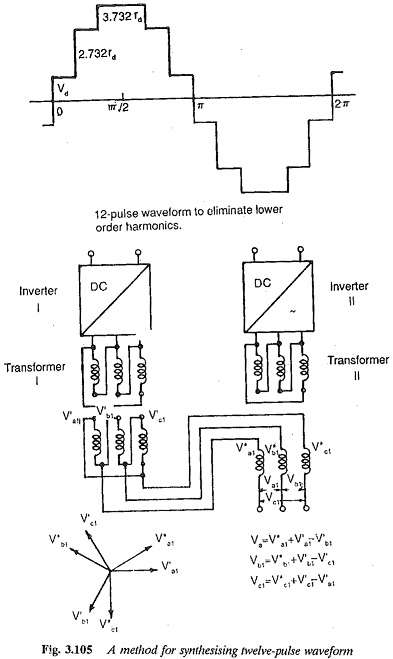The phase control of line commutated cycloconverters provides a variable voltage. The firing angle is varied over the period of the input voltage. The output voltage is made up of segments of the input voltage. The voltage control is possible in the inverter itself. Because of the phase control, the power factor is poor. A cycloconverter can be used to interconnect two three phase systems (Fig. 3.106).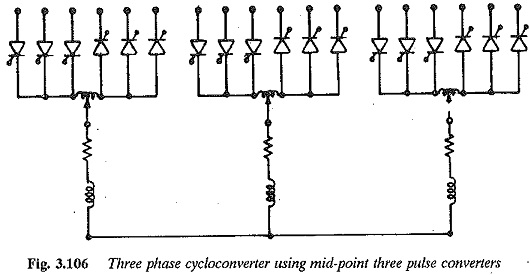The firing angle is so varied that the output voltage has a small distortion and is nearly sinusoidal. The load inductance further reduces the harmonic content in the current. The utilisation of the thyristors is poor at low frequencies. The cycloconverter allows power flow in both directions at any power factor of the load. The converter has a complex control system, and the output frequency can be varied from 0-1/2 input frequency. They are normally developed for 400 MHz, so that the output frequency is in the range 0-200 Hz.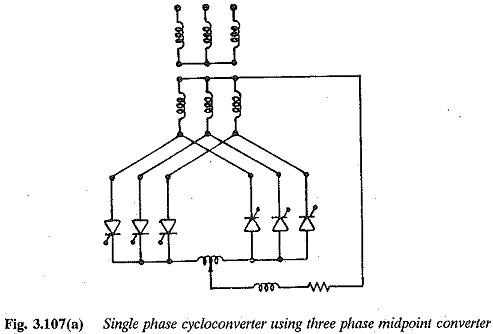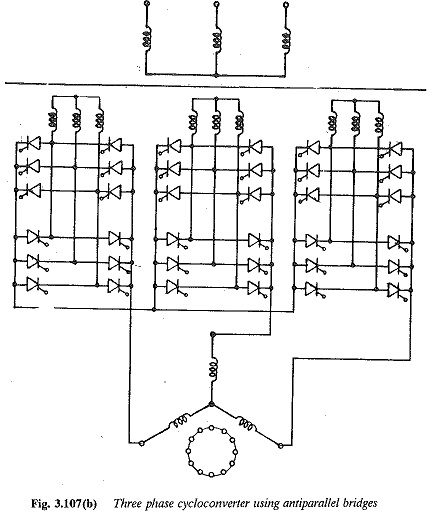The disadvantages of a poor power factor and a limited frequency range can be eliminated if forced commutation is employed. In this method, each converter has antiparallel three phase bridges provided with a common control circuit. The circuit details are given in Fig. 3.108.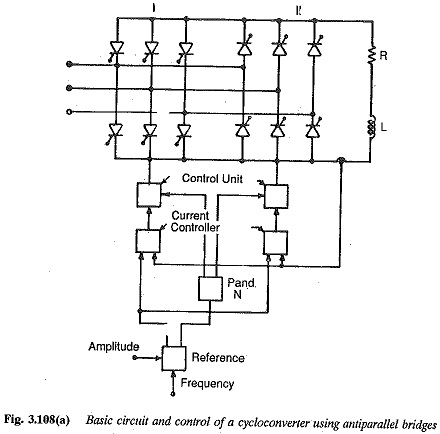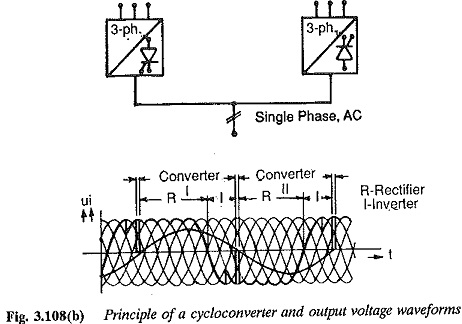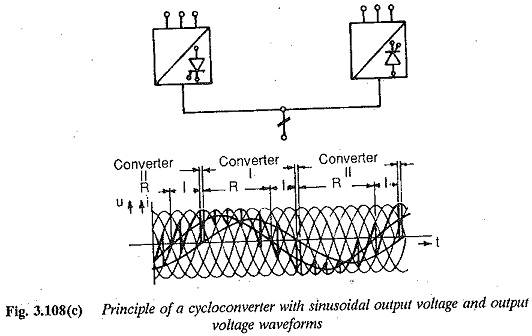The main features of cycloconverters can be summarised as follows:

1. Voltage control can be achieved in the converter itself.

2. A cycloconverter functioning by means of line commutation has poor p.f. and limitation of output frequency. Forced commutation can be employed to improve the p.f. and working range of frequencies. How­ever this introduces switching losses.

3. It is capable of power transfer in both directions between the source and the load. It can feed power to a load at any p.f. Regeneration is inherent over the complete frequency range.

4. It delivers high quality sinusoidal output waveforms at all operating

5. It requires many thyristors, which offsets the advantage of line commutation­ However, no shut down is required if a thyristor fails. Output can be made available without any interruption, albeit with a slightly distorted waveform.

The above discussion of cycloconverter shows that an a.c. motor (syn­chronous or induction), when fed from a cycloconverters will have a very smooth low speed operation with least torque ripple. Four quadrant opera­tion of the motor is straight forward as the cycloconverter allows power flow in either direction. Thus the cycloconverter is very attractive for feeding low speed, large power reversible ac motor drives.

Scroll to Top